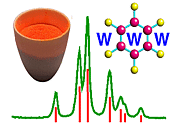Screw Symmetry III. Helical Screw Axes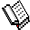Course Material Index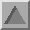Section Index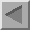Previous Page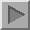Next Page

Helical Screw Axes

The two-one axis illustrated previously is not the only type of screw axis that can be found within a crystal structure. A threefold rotation axis, for example, may be combined with either a translation of 1/3 or 2/3 of the repeat distance along the axis direction. These two types of screw axis are referred to as "three-one" and "three-two" axes, and have the crystallographic notation 31 and 32, respectively.

In contrast to two-one screw axes, three-one and three-two axes possess a sense of handedness. Looking down the three-one axis, then the rotation is seen as 120° anticlockwise and the translation is 1/3 of the repeat distance (A to D) up the axis (as shown by the black arrows). This is illustrated in the figure below where the length of the horizontal black arrow corresponds to a translation length of 1/3 of the repeat distance along the axis direction. Starting with the red atom (A) on the far left of the diagram, you should be able to follow its movement along the axis as a result of repeated action of the three-one screw symmetry operator (A to B to C to D to E to F to G). The movement corresponds to that of a right-handed helical screw as shown by the blue ribbon. Note that it takes three times 31 to reach the middle (and equivalent) lattice position (D).

 31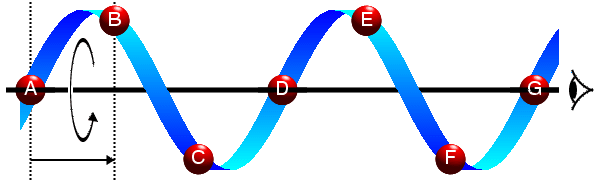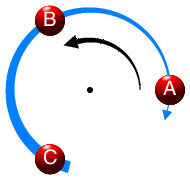View perpendicular to axis View down axis

Contrast this with the second figure showing a three-two axis where the rotation is still 120° anticlockwise, but the translation is now 2/3 of the repeat distance (as shown by the black arrows). The length of the horizontal black arrow corresponds to a translation length of 2/3 (A to C) of the repeat distance (A to D) along the axis direction. If the three-two screw axis symmetry operator is repeated twice, then the total rotation about the axis is 240° anticlockwise and the net translation along the axis direction is 4/3 (A to C to E). Due to lattice translational symmetry (E to B) there is also an equivalent lattice point (B) corresponding to a rotation of 240° and 1/3 translation along the axis direction. Thus a three-two screw axis corresponds to a left-handed helical screw (A to B to C to D to E to F to G).
 32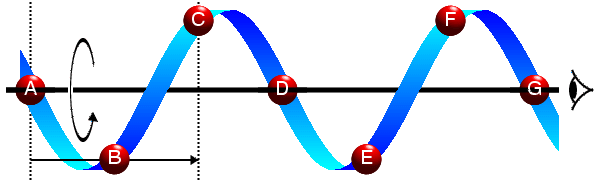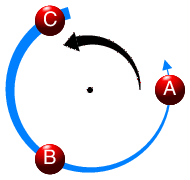View perpendicular to axis View down axis

Note that if you put a horizontal mirror perpendicular to the page in between the two figures, the two helices are mirror images of each other.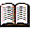The majority of the table is reference material.

In general, there exist crystallographic screw axes of the generic type n sub m, where n is the order of rotation, i.e. 2, 3, 4, or 6, m/n is the translation along the axis, and m < n, as shown in the table below:

OrderScrew axes
221
331, 32
441, 42, 43
661, 62, 63, 64, 65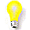Four-one (and six-one) and four-three (and six-five) screw axes correspond to right-handed and left-handed helices, respectively. Like the two-one screw axis, both the four-two and six-three axes have no sense. What is the sense of a six-two axis?Course Material IndexSection IndexPrevious PageNext Page
 © Copyright 1995-2006.  Birkbeck College, University of London. Author(s): Jeremy Karl Cockcroft Huub Driessen